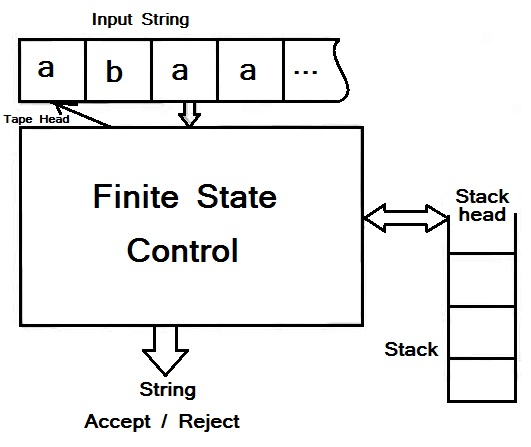Pushdown Automata Informal Definition:

The pushdown automaton is in essence a nondeterministic finite automata with ε-moves permitted and one additional stack capability on which it can store a string of “stack symbols”. The presence of a stack means that, unlike the finite automaton, the pushdown automaton can remember an infinite amount of information. However, unlike a general-purpose computer, which also has the ability to remember arbitrarily large amount of information, the pushdown automaton can only access the information on its stack in a LIFO (last-in-first-out) way.

Also Read: Pushdown automata introduction and requirement

There are languages that could be recognized by some computer program, but are not recognizable by any pushdown automaton. In fact, pushdown automata recognize all and only the context-free language. While there are many languages that are context-free, including some we have seen that are not regular languages, there are also some simple-to-describe languages hat are not context-free. For an example of non-context free language is M = {anbncn | n ≥ 1}, The set of strings consisting of equal groups of a’s, b’s and c’s.Figure: A pushdown automata is essentially a finite automata with a stack data structure

The informal way of pushdown automata representation is as represented in the above figure. Which consist a “Finite-state control” reads inputs, one symbol at a time. The pushdown automata are allowed to observe the symbol at the top of the stack and to base its transaction on its current state, the input symbol, and the symbol at the top of the stack. Alternatively, it may make a “spontaneous” transition, using ε as its input instead of an input symbol. In one transition, the pushdown automata:

• Consumes from the input the symbol that it uses in the transition. If ε is used for the input, then no input symbol is consumed.
• Goes to a new state, which may or may not be the same as the previous state.

Replace the symbol at the top of the stack by any string. The string could be ε, which corresponds to a pop of the stack. It could be the same symbol that appeared at the top of the stack previously; i.e. no change to the stack is made. If could also replace the top stack symbol does not push or pop it. Finally, the top stack symbol could be replaced by two or more symbols, which has the effect of (possibly) changing the top stack symbol, and then pushing one or more symbol onto the stack.

Pushdown Automata Formal Definition:

The formal notation for a pushdown automata (PDA) involves seven tuples. We write the specification of a PDA ‘M’ as follows;

M = (Q, ∑, Γ, δ, q0 , z0, F)

These tuples have the following meaning;

1. Q: A finite set of states, like the states of a finite automation.
2. ∑: A finite set of input alphabet/ symbols, also analogous to the corresponding component of a finite automaton.
3. Γ: A finite stack alphabet. This touple, which has no finite-automaton analog, it the set of symbols that we are allowed to push onto the stack.
4. δ: The transaction function. As for a finite automation, δ governs the behavior of the automaton. Formally, δ takes as argument a triple δ(q, a, X), where;
1. q is a state in Q.
2. a is either an input symbol in ∑ or a = ε, the empty string, which is assumed not to be an input symbol.
3. X is a stack symbol, that is a member of Γ.

Basically the complete transaction function of pushdown automata is as;

δ: (Q x (Σ ∪{ε}) x X) → (p, γ)

Here the output of δ is a finite set of pair (p, γ), where p is the new state, and γ is the string of stack symbols that replaces X at the top of the stack. For instance, if γ = ε, then the stack is popped, if γ = X, then the stack is unchanged, and if γ = YZ, then X  is replaced by Z and Y is pushed onto the stack.

1. q0: The initial state. The PDA is in this state before making any transitions.
2. z0: The stack start symbol. Initially, the PDA’s stack consists of one instance of this symbol, and nothing else.
3. F: The set of final states or accepting states.

This site uses Akismet to reduce spam. Learn how your comment data is processed.